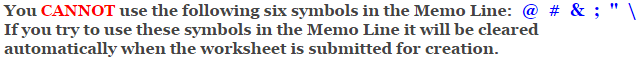# Pythagorean Theorem Worksheets

## Pythagorean Theorem Problems Worksheets

This Pythagorean Theorem Problems Worksheet will produce problems for practicing solving the lengths of right triangles.

You may choose the type of numbers and the sides of the triangle.

### Language for the Pythagorean Theorem Worksheet

 English German Albanian Spanish Swedish Italian French Turkish Polish Norwegian

### Memo Line for the Pythagorean Theorem Worksheet

You may enter a message or special instruction that will appear on the bottom left corner of the Pythagorean Theorem Worksheet.### Pythagorean Theorem Worksheet Answer Page

Now you are ready to create your Pythagorean Theorem Worksheet by pressing the Create Button.

 Recommended Videos

If You Experience Display Problems with Your Math Worksheet